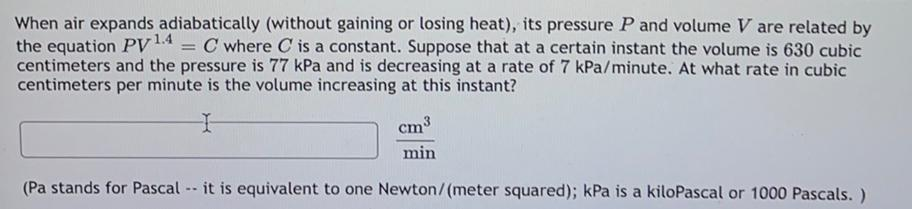Question:

# When air expands adiabatically (without gaining or losing heat), its pressure P and volume V are related by the equation Py 1.4When air expands adiabatically (without gaining or losing heat), its pressure P and volume V are related by the equation Py 1.4 = C where C is a constant. Suppose that at a certain instant the volume is 630 cubic centimeters and the pressure is 77 kPa and is decreasing at a rate of 7 kPa/minute. At what rate in cubic centimeters per minute is the volume increasing at this instant? cm3 min (Pa stands for Pascal -- it is equivalent to one Newton/meter squared); kPa is a kiloPascal or 1000 Pascals. ) a